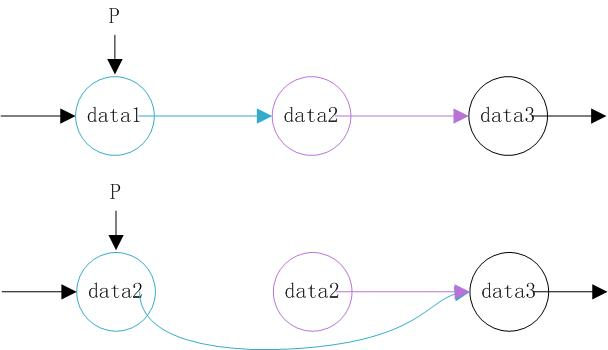# 一个帅气的链表节点删除引起的错误```/* file list.h */
#ifndef __LIST_H__
#define __LIST_H__
#include <stdlib.h>

};

#define list_entry(ptr, type, member) \
container_of(ptr, type, member)
#define container_of(ptr, type, member) ({ \
const typeof( ((type*)0)->member ) *__mptr = (ptr); \
(type *)( (char *)__mptr - offsetof(type, member));})

#define for_each_node(list, name)                    \
for (name = list->next; name != list; name = name->next)

{
new->next = prev->next;
prev->next = new;
}

static inline void list_del(struct list_head *p)
{
*p = *p->next;
}

static inline void list_delete_if(struct list_head *list,
{
for_each_node(list, p) {
if (f(p)) {
list_del(p);
nfree(t);
}
}
}

static inline void list_travel(struct list_head *list,
{
for_each_node(list, p)
f(p);
}
#endif /* __LIST_H__ */
```
```/* file main.c */
#include <stdio.h>
#include <malloc.h>
#include "list.h"

struct A {
int val;
};

{
printf("node->val=%d,", list_entry(e, struct A, list)->val);
}

{
return list_entry(e, struct A, list)->val == 1;
}

{
free(list_entry(e, struct A, list));
}

int main()
{

struct A *node0 = (struct A*)malloc(sizeof(struct A)),
*node1 = (struct A*)malloc(sizeof(struct A));

node0->val = 1; node1->val = 2;

list_travel(&list_of_a, print_node);
putchar('\n');

printf("delete node->val=2\n");
list_delete_if(&list_of_a, val_is_2_p, node_free);

list_travel(&list_of_a, print_node);
putchar('\n');

return 0;
}
```

```static inline void list_del(struct list_head **p)
{
*p = (*p)->next;
}
```